RHESSI Detector Resolution

Resolution is the sum of a constant term (electronic resolution), a term proportional to the square root of energy (natural statistics of germanium electron/hole pairs), and a term proportional to energy (trapping).

resolution(E) = sqrt ( A^2. + (B*sqrt(E))^2. + (C*E)^2. )

The values of A, B, and C for each detector segment are read from a table of parameters vs dates (hessi_resol_vs_time.1000) and interpolated to the requested date.  The units of the resolution are FHWM in keV of a gaussian line.

The following plots show the calculated RHESSI detector FWHM resolution as a function of both time and energy.  These are the resolutions used in calculating the spectral response matrix in the RHESSI software.

PLOTS:

Front Segments at:   start of mission    2008    2016
Rear Segments at:    start of mission    2008    2016
Front Segment history at:    10 keV   150 keV
Rear Segment history at:   200 keV    15 MeV
Notes

Front Segments in 2002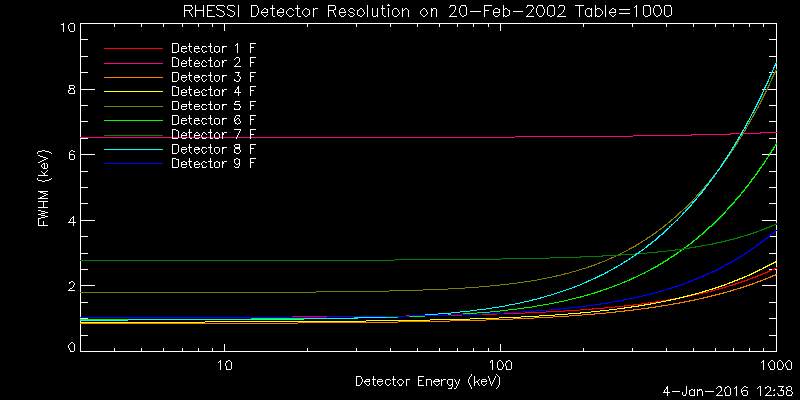IDL COMMAND:   hsi_plot_resolutions,time='20-feb-2002',xstyle=1, /png,filename='res_2002_front.png'

Front Segments in 2008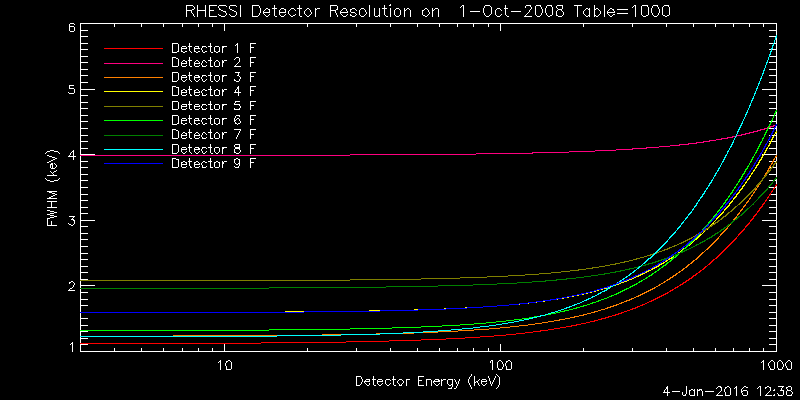IDL COMMAND:  hsi_plot_resolutions,time='1-oct-2008',xstyle=1, /png,filename='res_2008_front.png'

Front Segments in 2016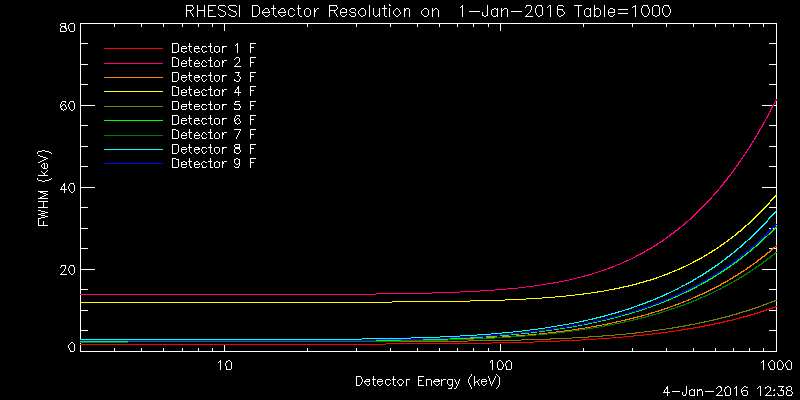IDL COMMAND:  hsi_plot_resolutions,time='1-jan-2016',xstyle=1, /png,filename='res_2016_front.png'

Rear Segments in 2002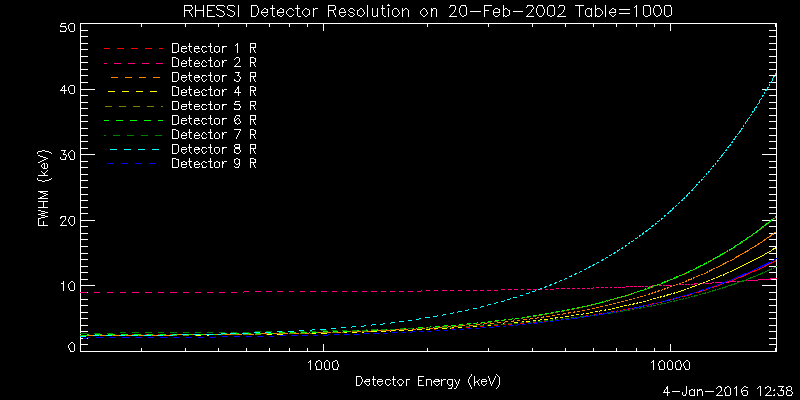IDL COMMAND:  hsi_plot_resolutions,/rear,xstyle=1, time='20-feb-2002', energy=findgen(1001)*20.+200, /png,filename='res_2002_rear.png'

Rear Segments in 2008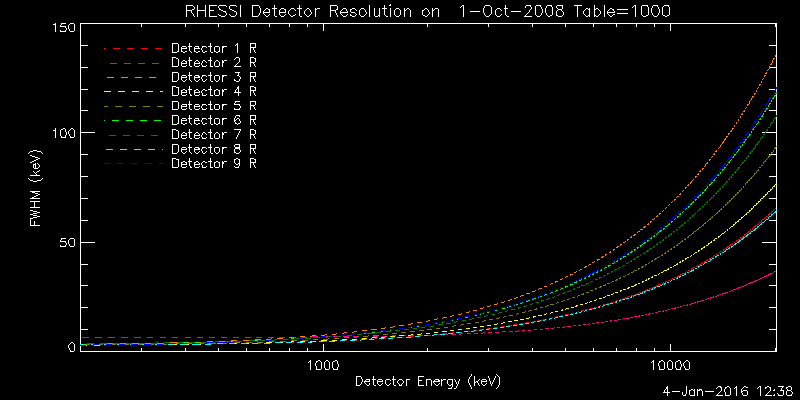IDL COMMAND:  hsi_plot_resolutions,/rear,xstyle=1, time='1-Oct-2008', energy=findgen(1001)*20.+200, /png,filename='res_2008_rear.png'

Rear Segments in 2016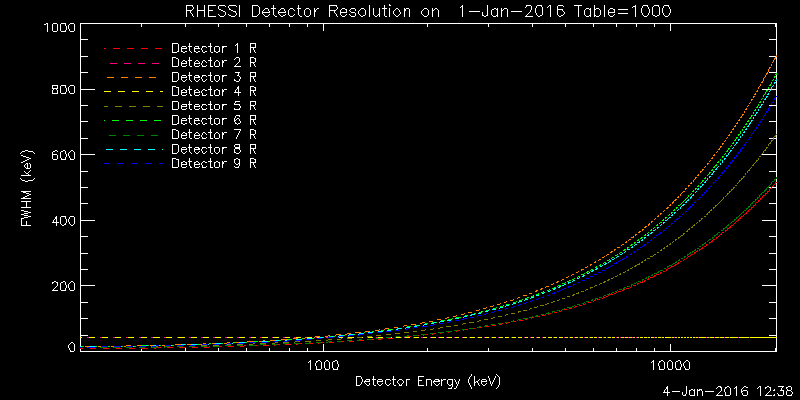IDL COMMAND:  hsi_plot_resolutions,/rear,xstyle=1, time='1-jan-2016', energy=findgen(1001)*20.+200, /png,filename='res_2016_rear.png'

Front Segment history at 10 keV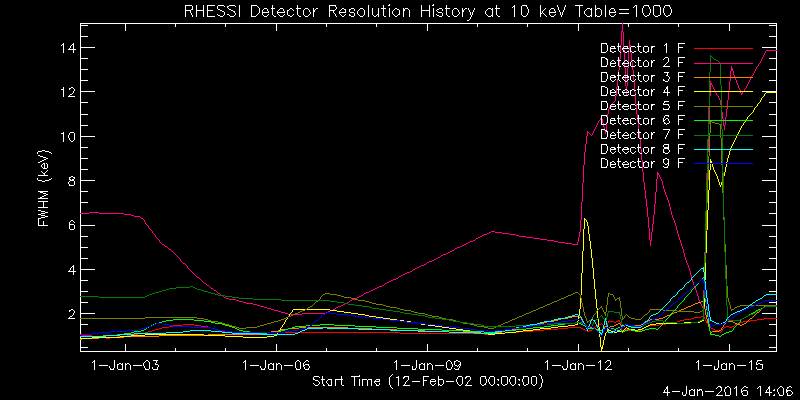IDL COMMAND:  hsi_plot_res_history,energy=10,ystyle=1,xstyle=1,/png,filename='res_history_10keV_front.png'

smaller y range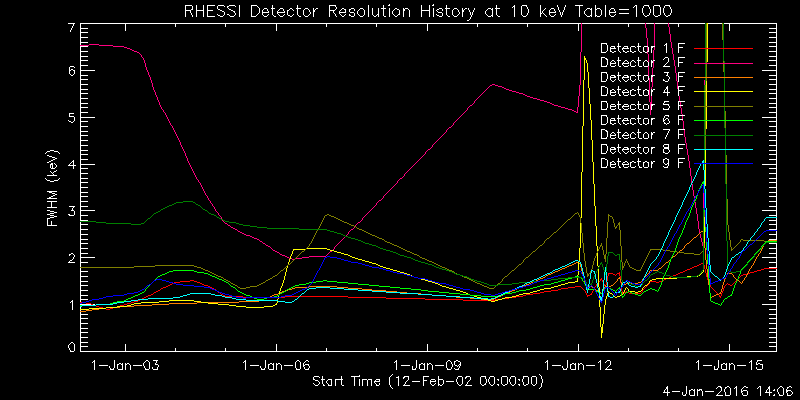Front Segment history at 150 keV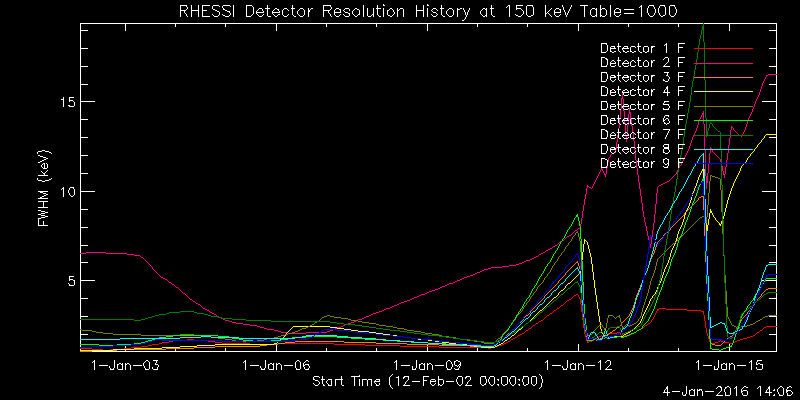IDL COMMAND:  hsi_plot_res_history,energy=150,ystyle=1,xstyle=1,/png,filename='res_history_150keV_front.png'

smaller y range:Rear Segment history at 200 keV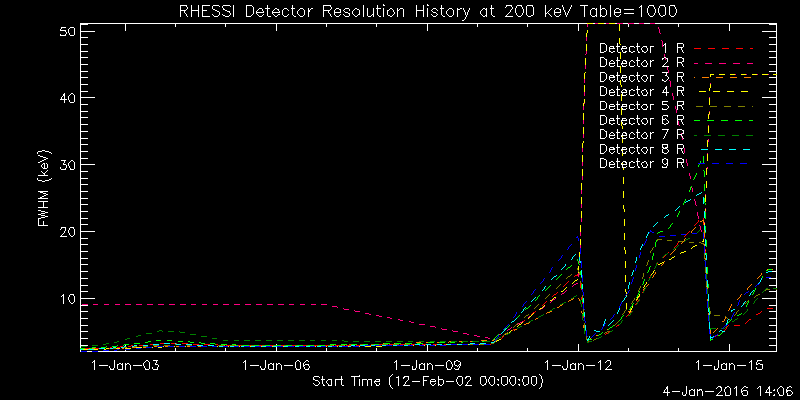IDL COMMAND:  hsi_plot_res_history,energy=200,/rear,xstyle=1,/png,filename='res_history_200keV_rear.png'

smaller y range: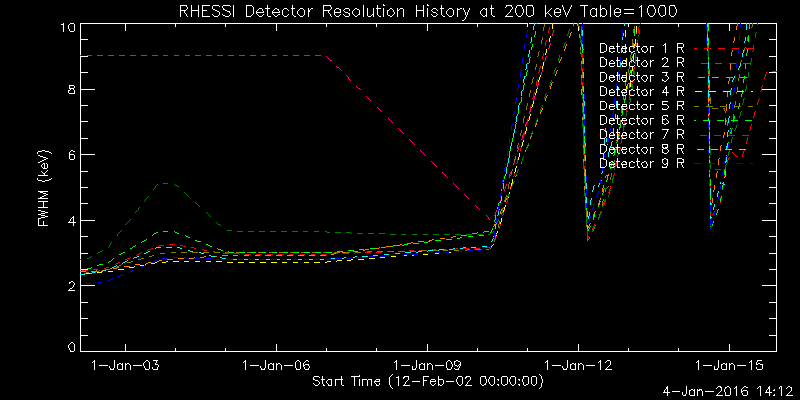Rear Segment history at 15 MeV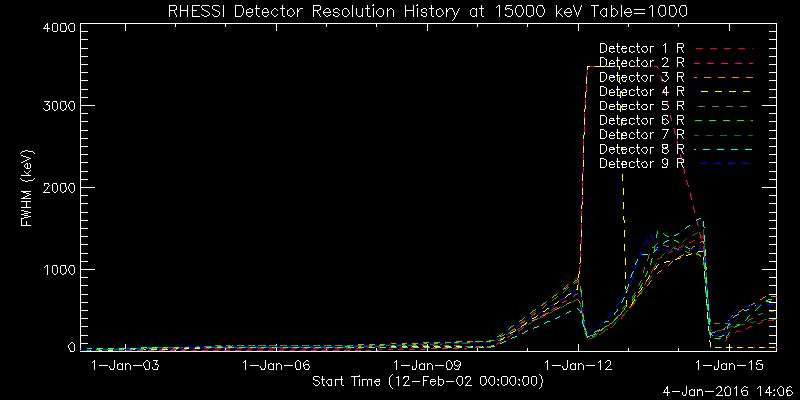IDL COMMAND:  hsi_plot_res_history,energy=15000,/rear,xstyle=1,/png,filename='res_history_15000keV_rear.png'

smaller y range: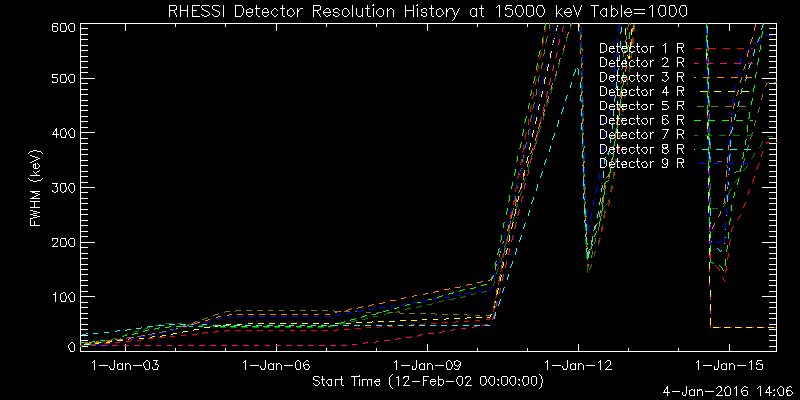• We do not understand the details of the resolution history plots, including the hump in the resolutions centered around  January 2004 and the sudden rise in Detector 4 Front Segment resolution at the beginning of 2006.
• The command to generate each plot is shown under the plot.  In an sswidl session, you can generate similar plots for any time and energy using the hsi_plot_resolutions and hsi_plot_res_history routines.

Last edited 04 January 2016 by Kim Tolbert